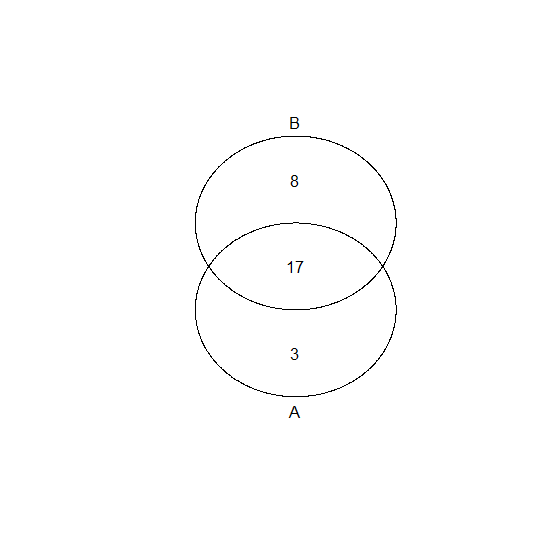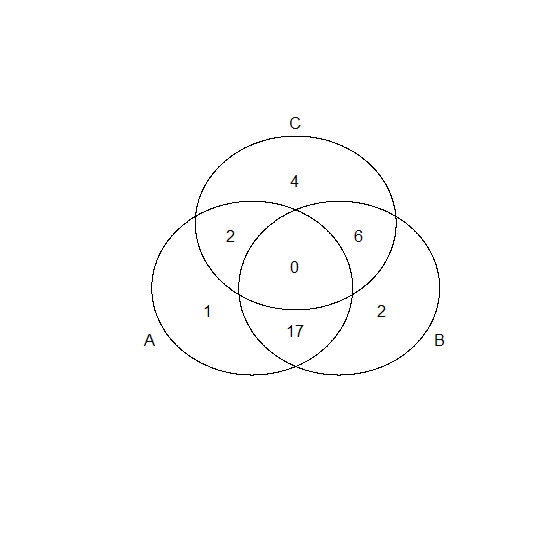# How to create a Venn diagram in R?

A Venn diagram helps to identify the common and uncommon elements between two or more than two sets of elements. This is also used in probability theory to visually represent the relationship between two or more events. To create a Venn diagram in R, we can make use of venn function of gplots package.

## Example

Consider the below vectors

x<-c(rep(c(1,2,3),times=c(4,5,8)),12,15,20)
y<-c(1:10,25)

install.packages("gplots")
library(gplots)

Creating the Venn diagram for x and y −

venn(list(x,y))

## OutputIf we have three variables then we will just add the third variable in venn function as shown below −

z<-c(5:15,21)
venn(list(x,y,z))

## Output